## Logarithmic SpiralA curve whose equation in Polar Coordinates is given by(1)

whereis the distance from the Origin,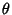is the angle from the-axis, andandare arbitrary constants. The logarithmic spiral is also known as the Growth Spiral, Equiangular Spiral, and Spira Mirabilis. It can be expressed parametrically using(2)

which gives(3)(4)

The logarithmic spiral was first studied by Descartesin 1638 and Jakob Bernoulli.Bernoulli was so fascinated by the spiral that he had one engraved on his tombstone (although the engraver did not draw it true to form). Torricelli worked on it independently and found the length of the curve (MacTutor Archive).

The rate of change of Radius is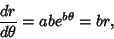(5)

and the Angle between the tangent and radial line at the point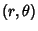is(6)

So, as,and the spiral approaches a Circle.

Ifis any point on the spiral, then the length of the spiral fromto the origin is finite. In fact, from the pointwhich is at distancefrom the origin measured along a Radius vector, the distance fromto the Pole along the spiral is just the Arc Length. In addition, any Radius from the origin meets the spiral at distances which are in Geometric Progression (MacTutor Archive).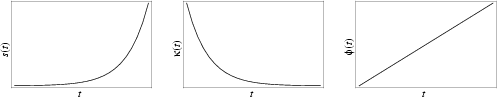The Arc Length, Curvature, and Tangential Angle of the logarithmic spiral are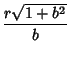(7)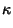(8)(9)

The Cesàro Equation is(10)

On the surface of a Sphere, the analog is a Loxodrome. This Spiral is related to Fibonacci Numbers and the Golden Mean.

References

Lawrence, J. D. A Catalog of Special Plane Curves. New York: Dover, pp. 184-186, 1972.

Lee, X. EquiangularSpiral.'' http://www.best.com/~xah/SpecialPlaneCurves_dir/EquiangularSpiral_dir/equiangularSpiral.html

Lockwood, E. H. The Equiangular Spiral.'' Ch. 11 in A Book of Curves. Cambridge, England: Cambridge University Press, pp. 98-109, 1967.

MacTutor History of Mathematics Archive. Equiangular Spiral.'' http://www-groups.dcs.st-and.ac.uk/~history/Curves/Equiangular.html.